# Selina Solutions Concise Maths Class 10 Chapter 7 Ratio and Proportions

Basic concepts of ratio and proportion studied in earlier classes are continued with more in-depth knowledge in this chapter. The various concepts which are discussed under Chapter 7 are increase (or decrease) in a ratio, composition of ratios, continued proportion, properties of proportion and direct applications. Our expert faculty at BYJU’S have created detailed solutions to the exercise wise problems to help students with their exam preparations. The solutions are designed in a simple stepwise manner to meet the understanding capacity of all students. Students aiming to improve their problem solving and build confidence over this chapter can take the help of Selina Solutions for Class 10 Mathematics Chapter 7 Ratio and Proportions free PDF, from the links available here.

## Selina Solutions Concise Maths Class 10 Chapter 7 Ratio and Proportions Download PDF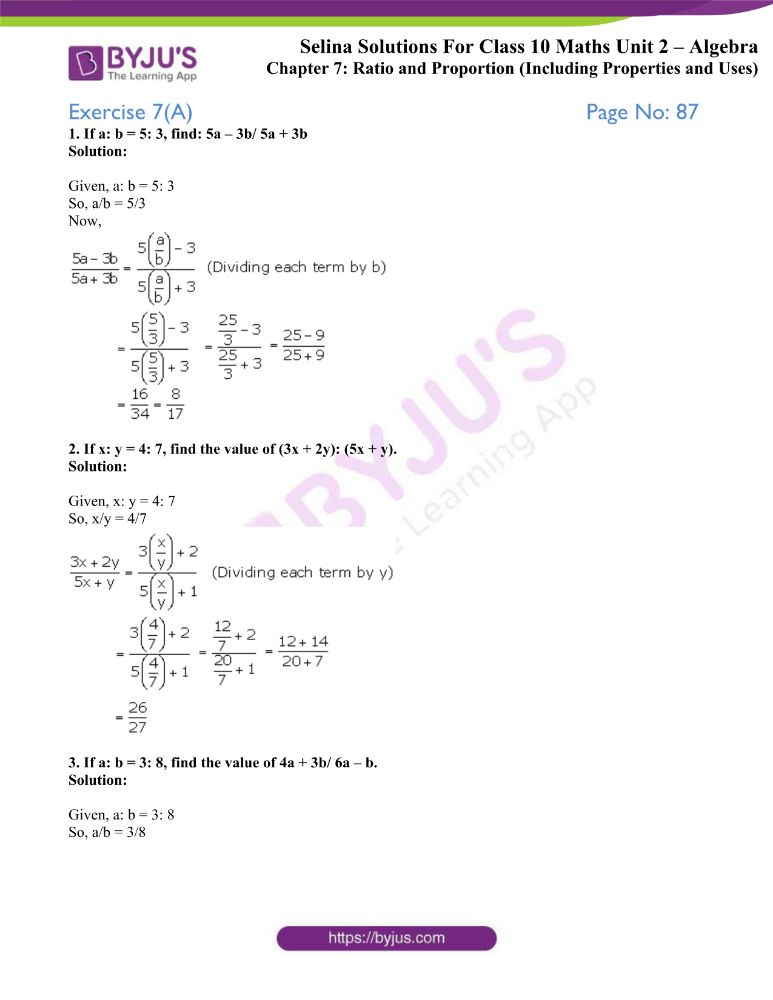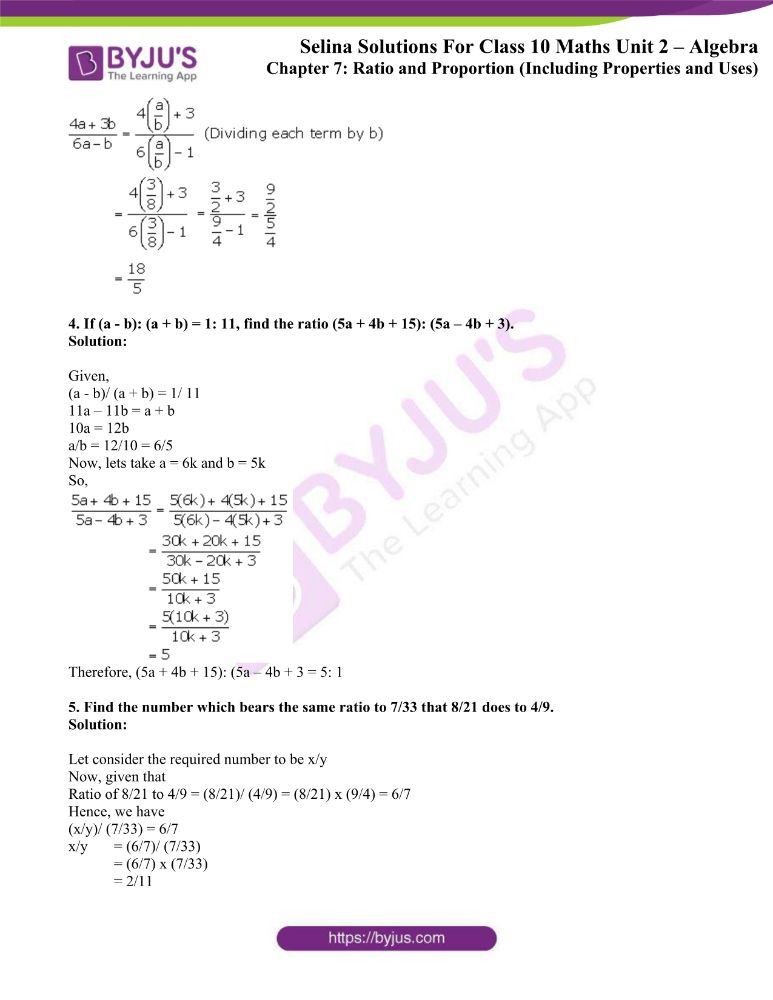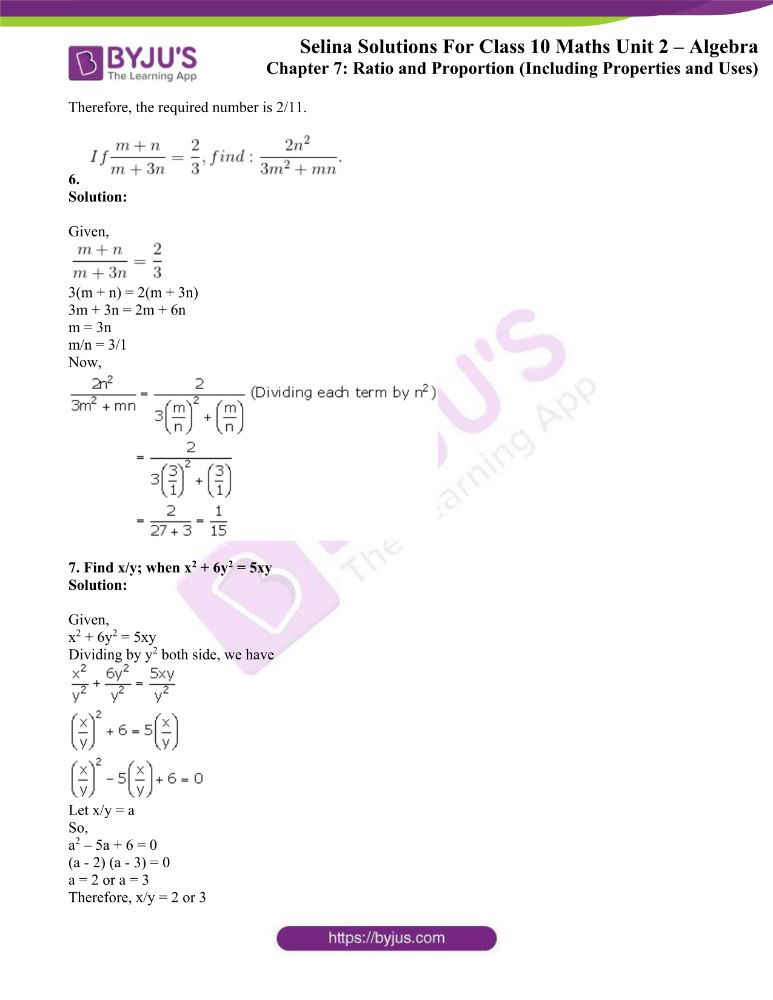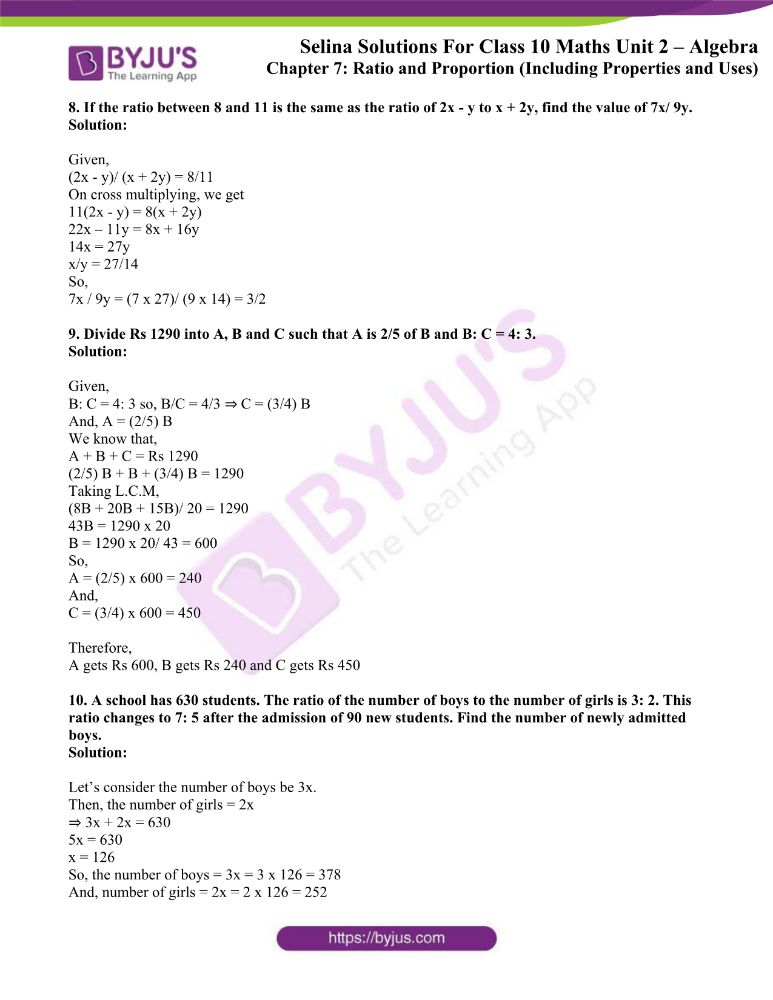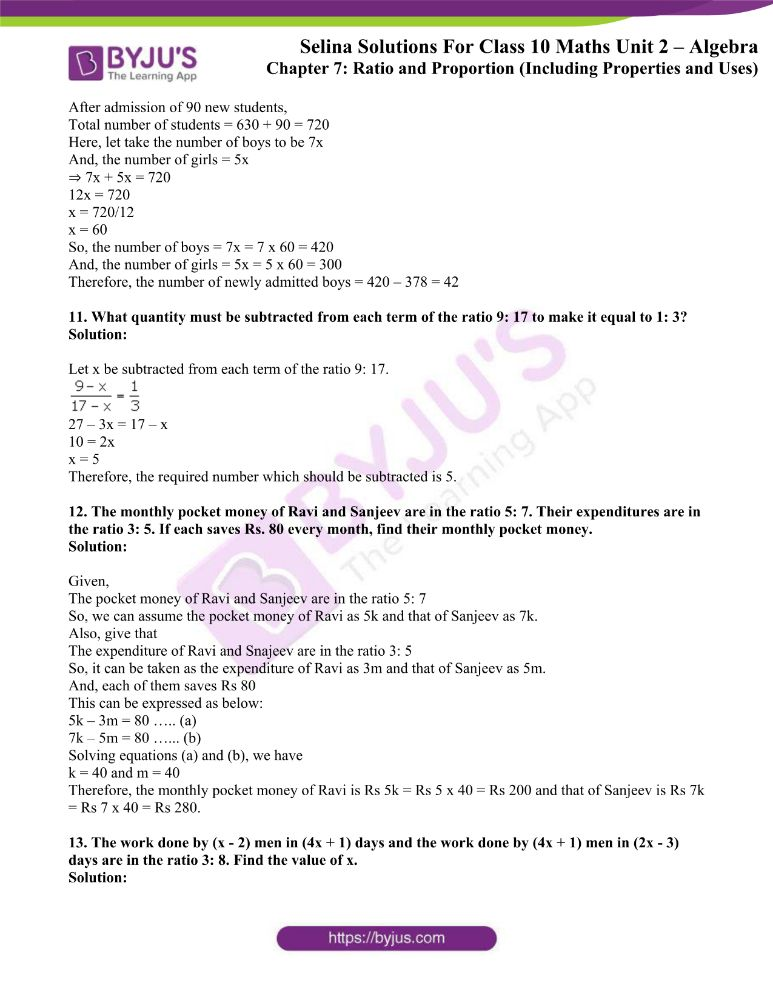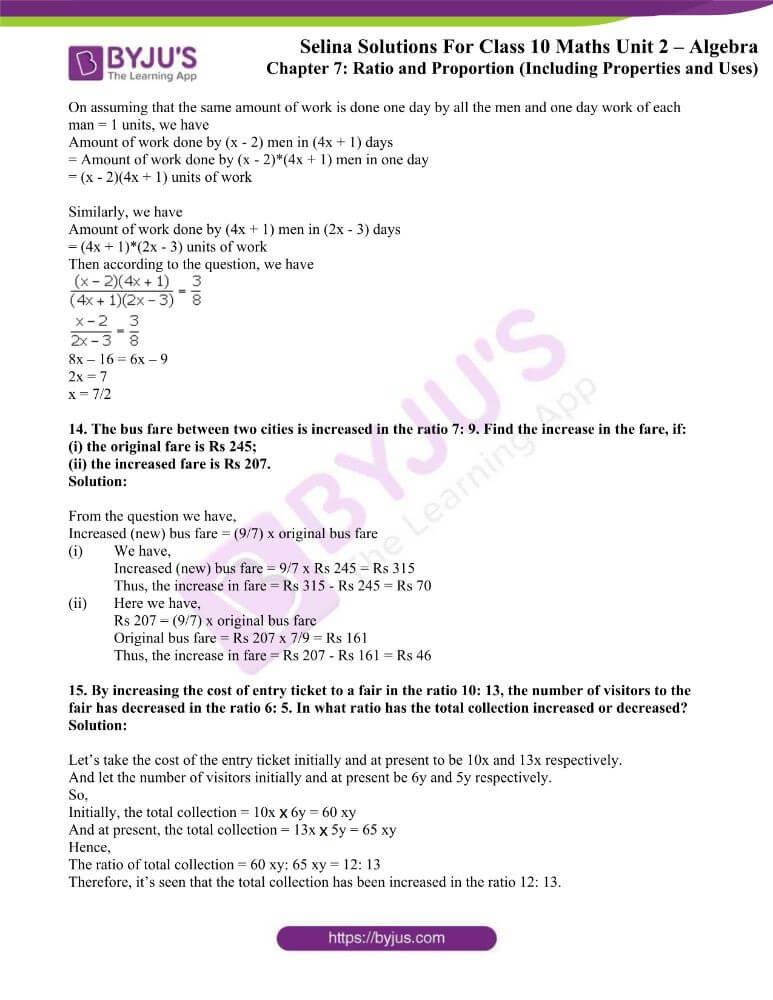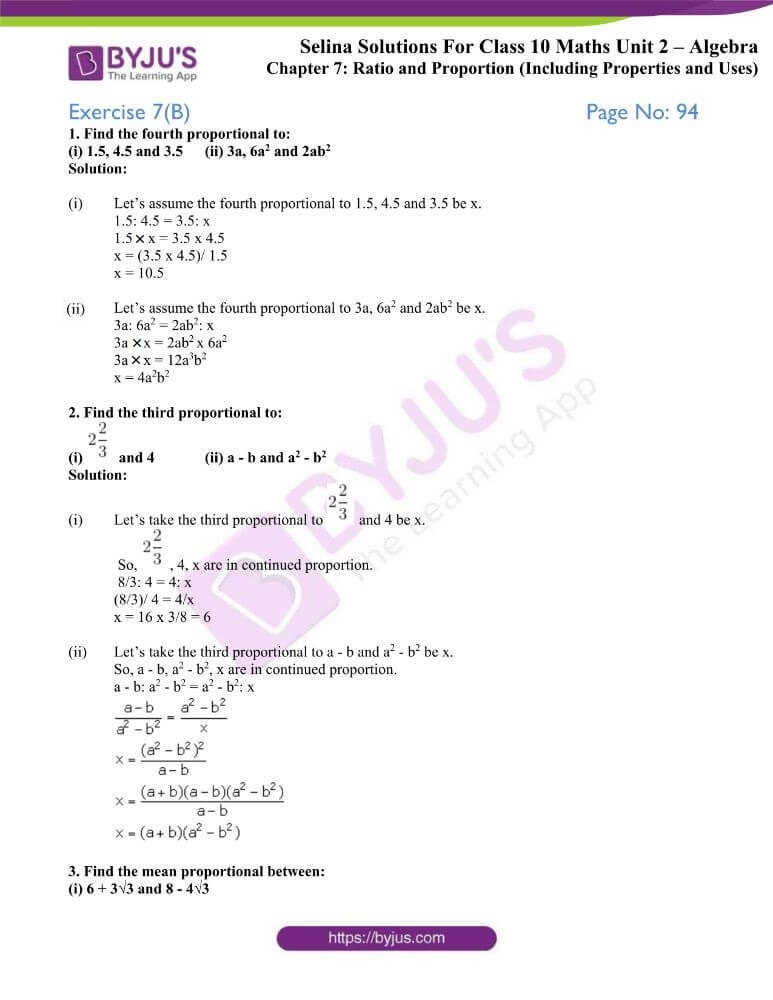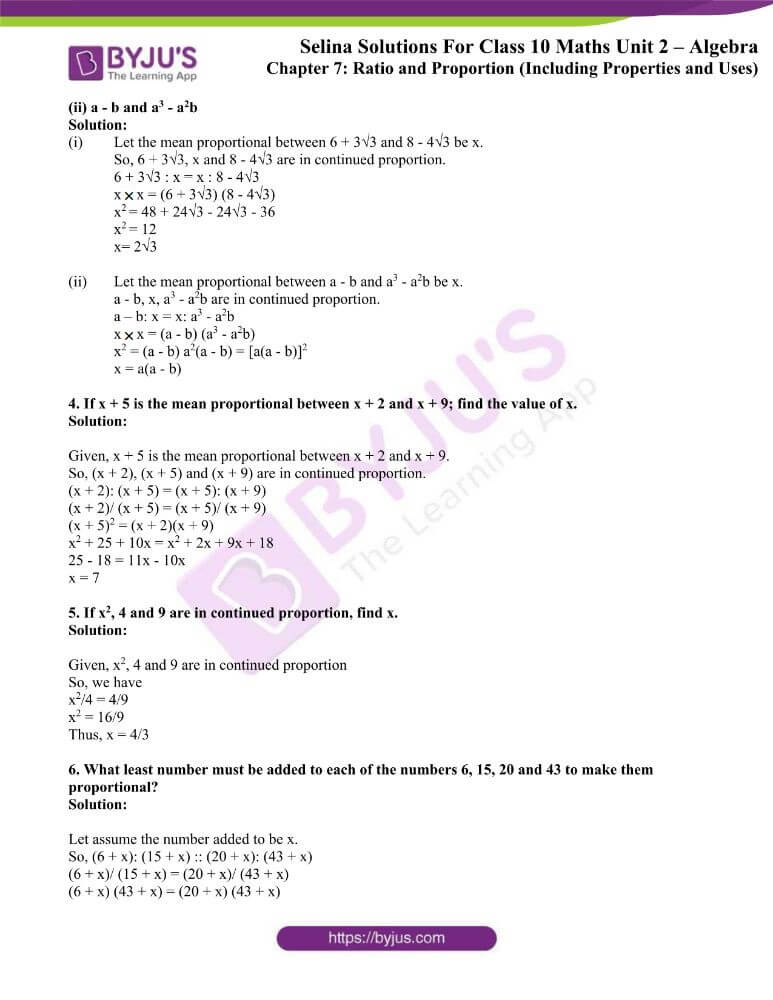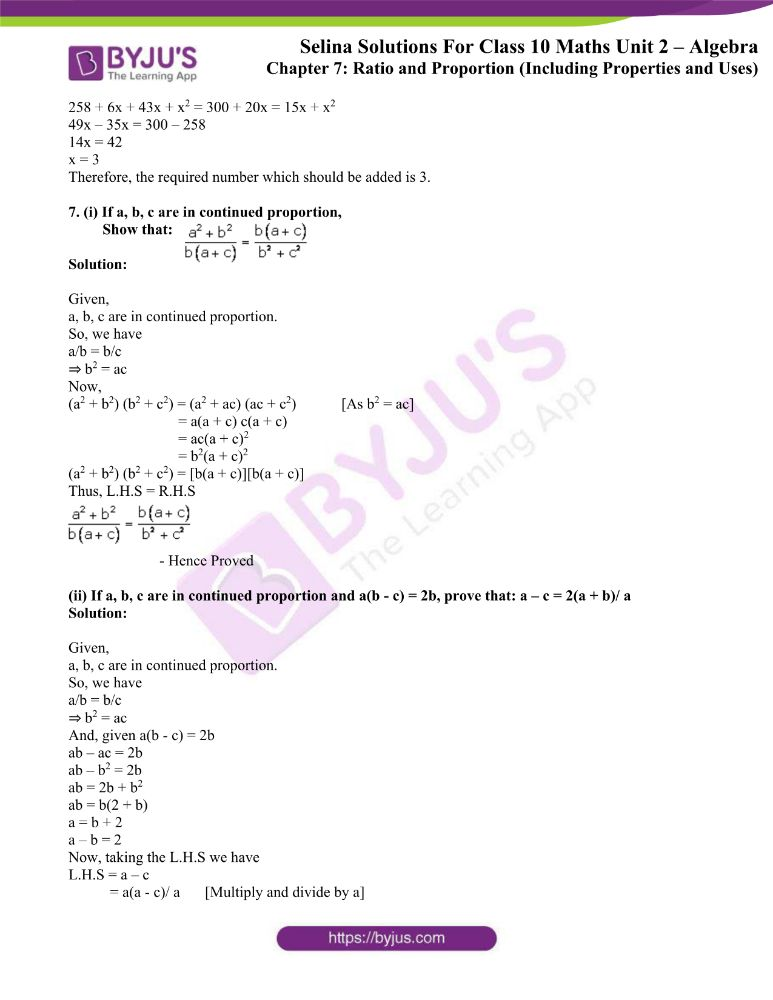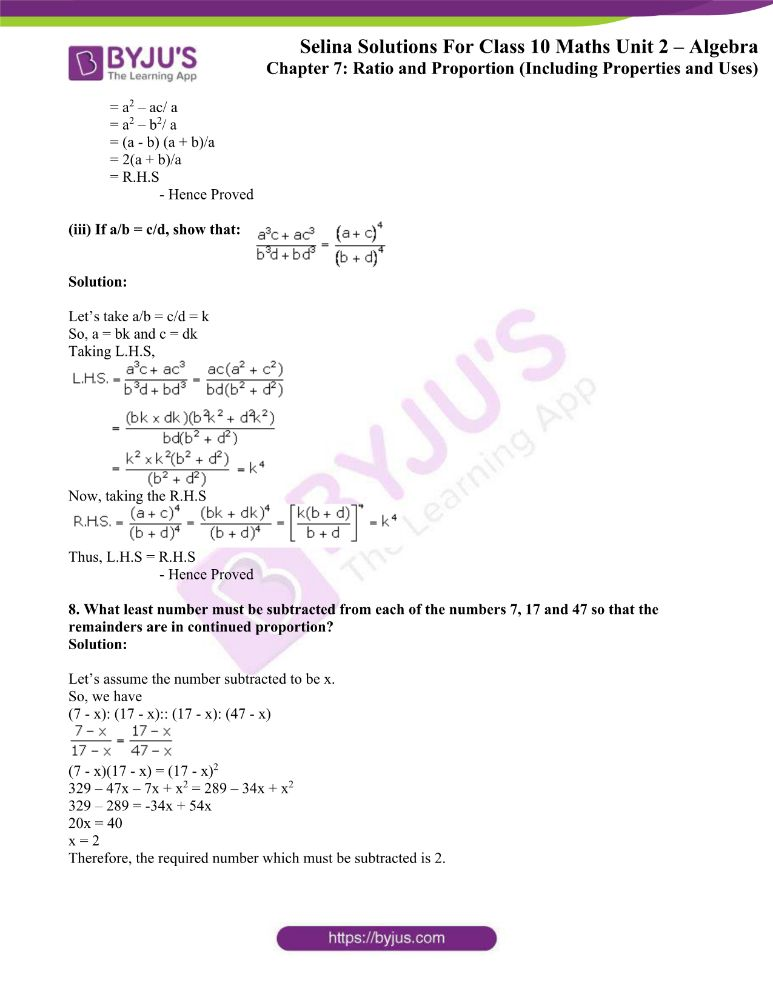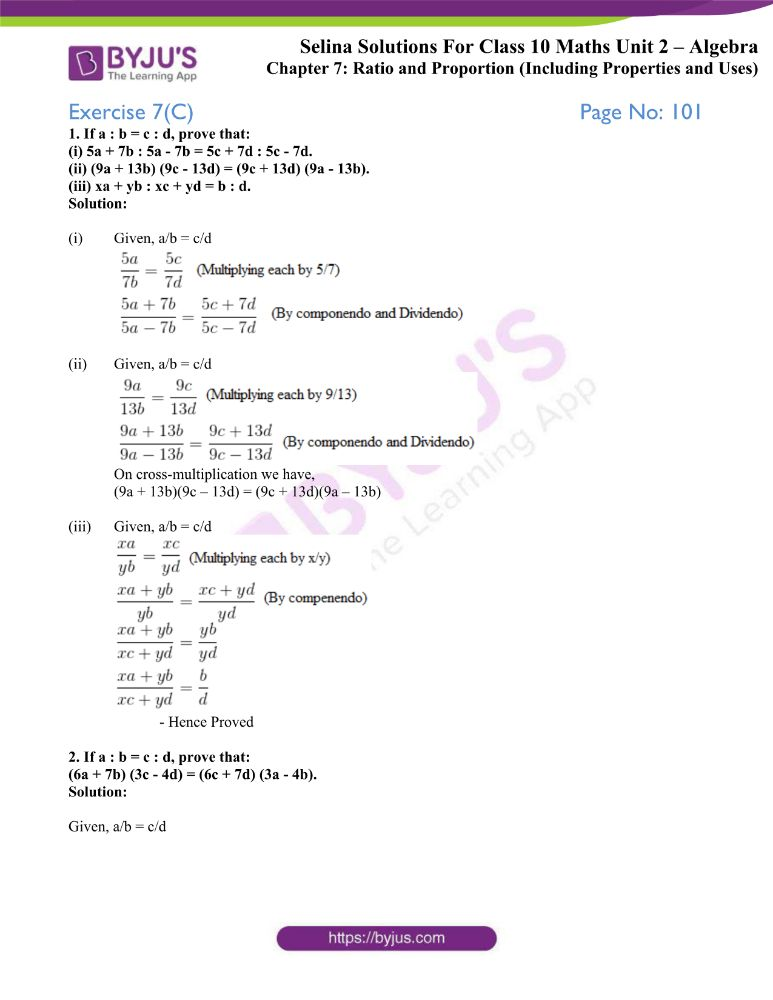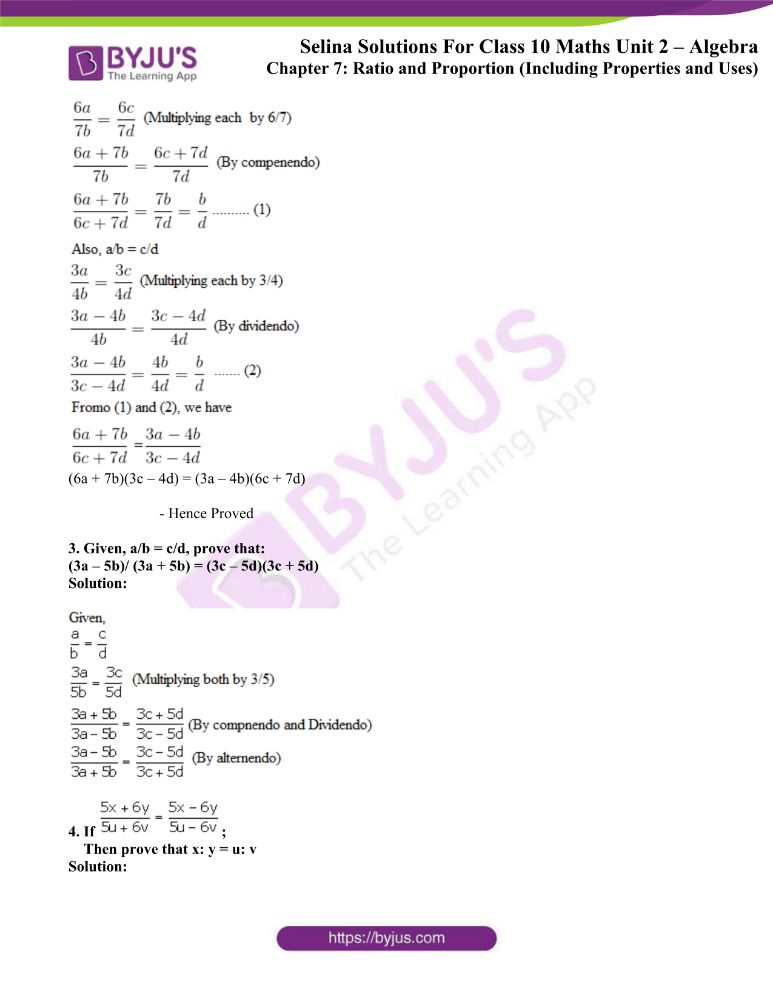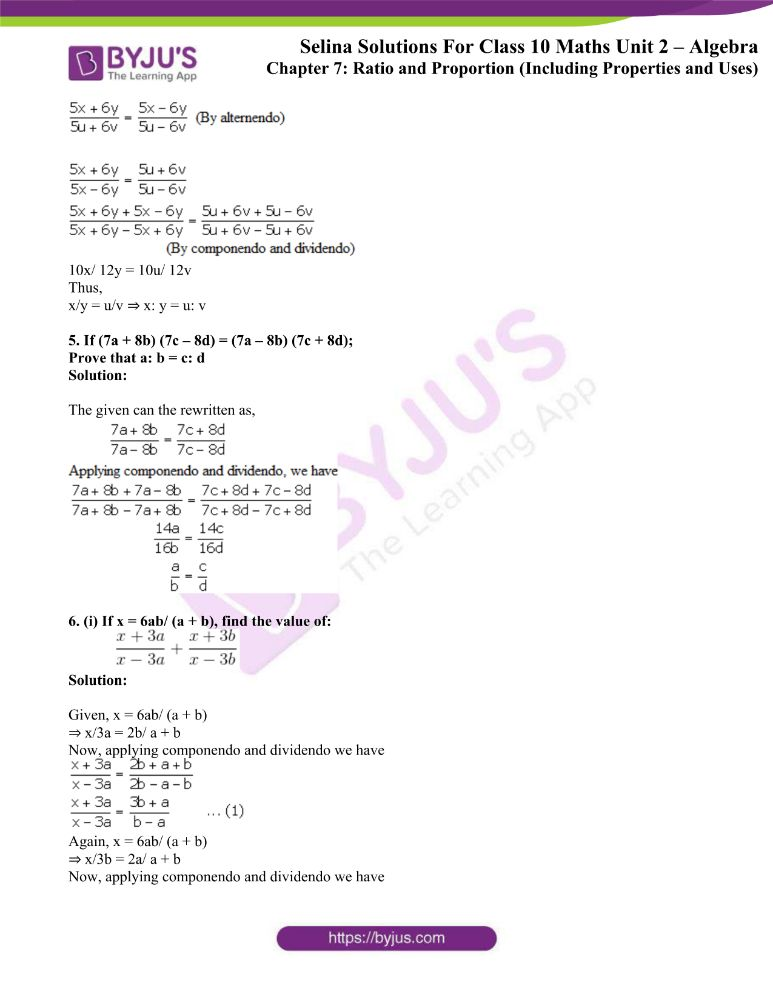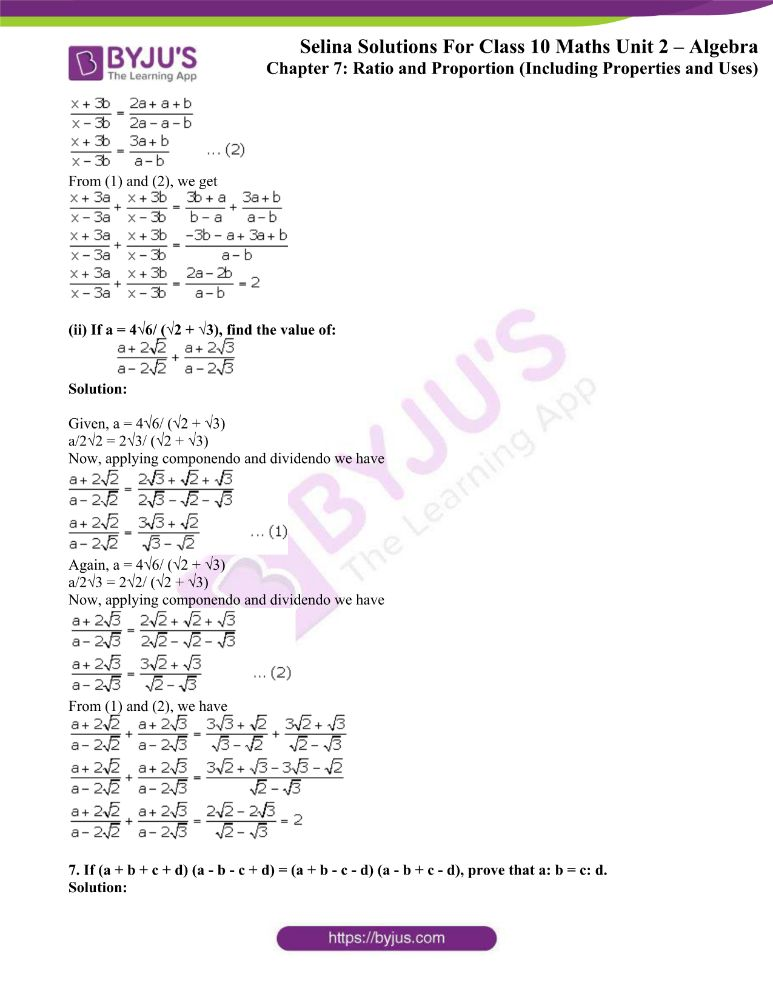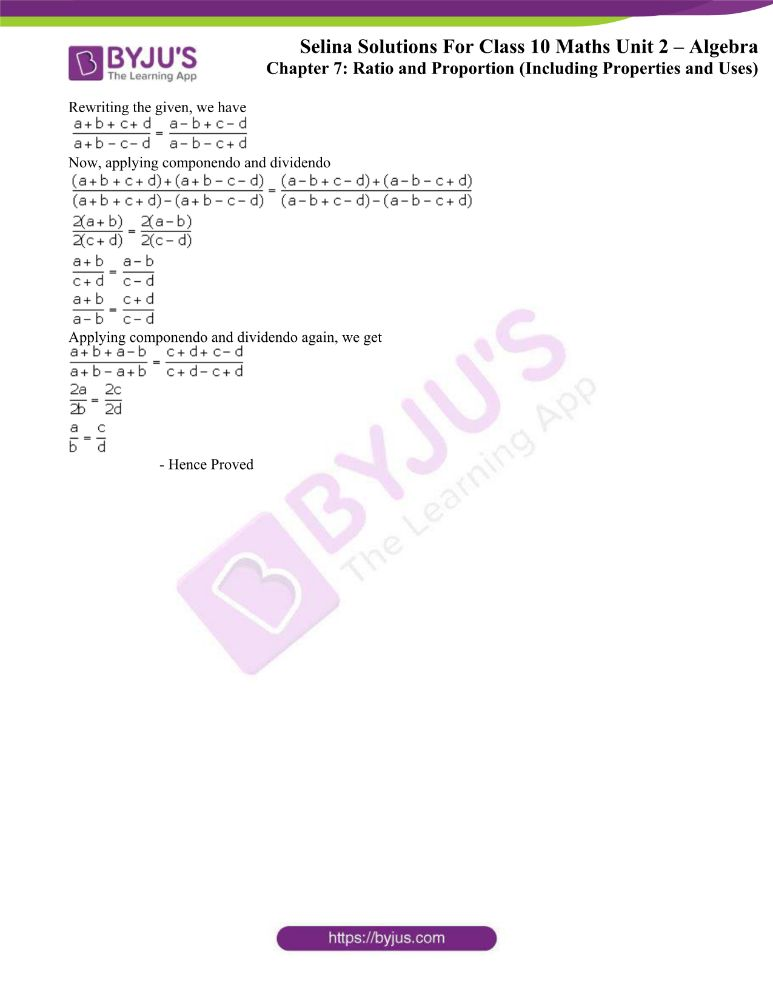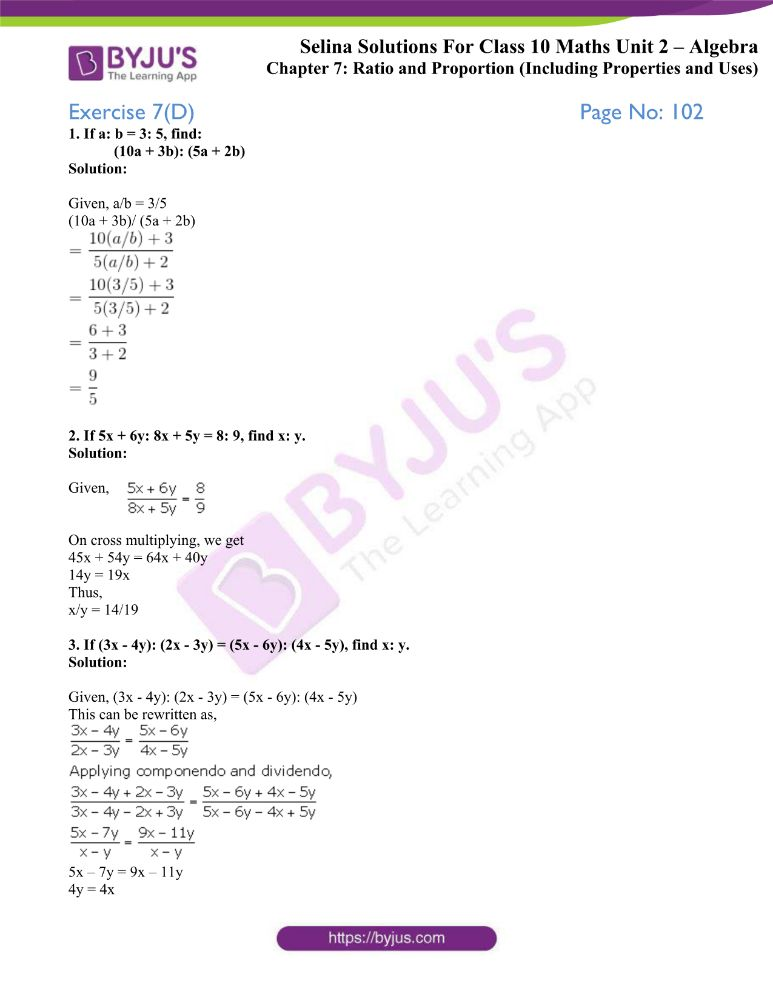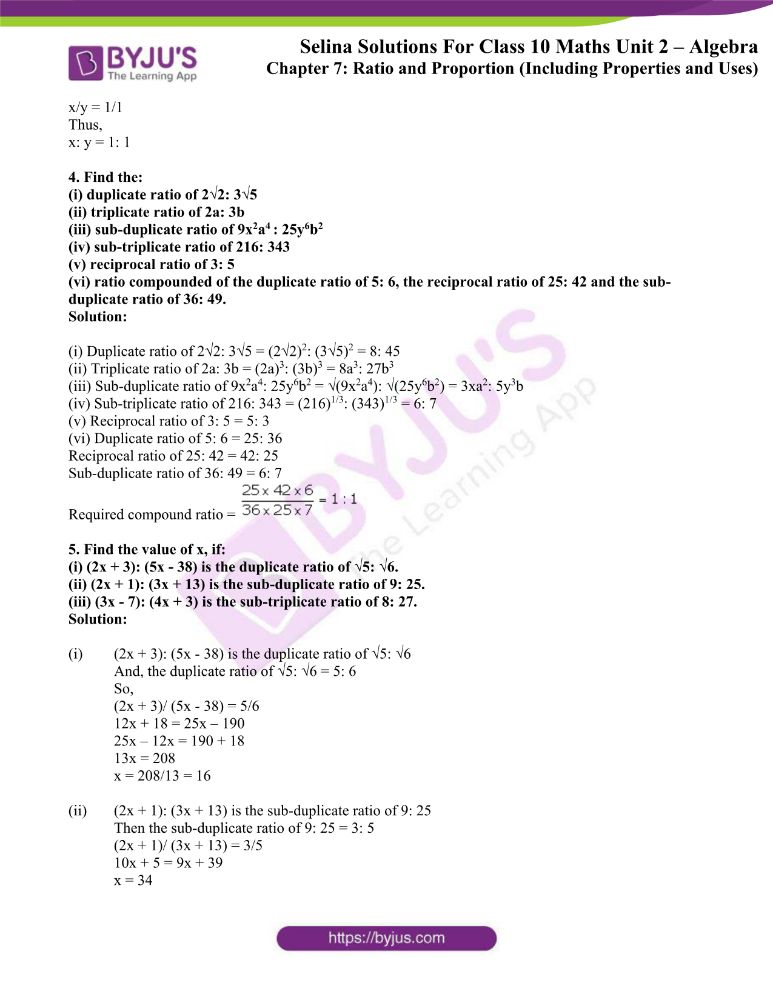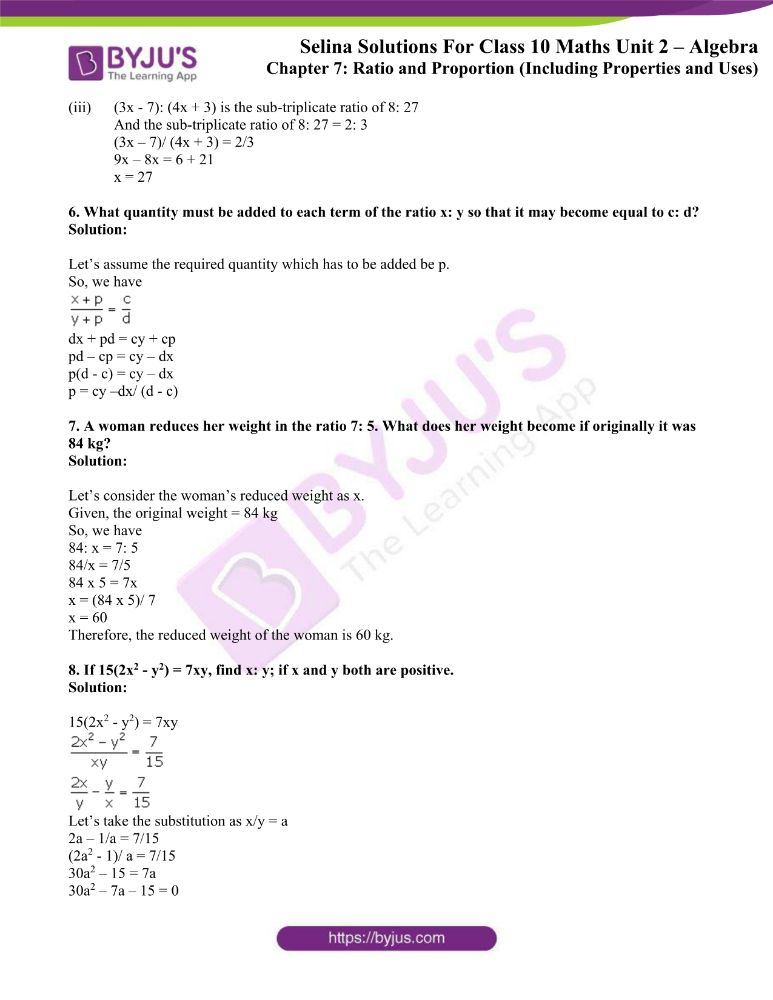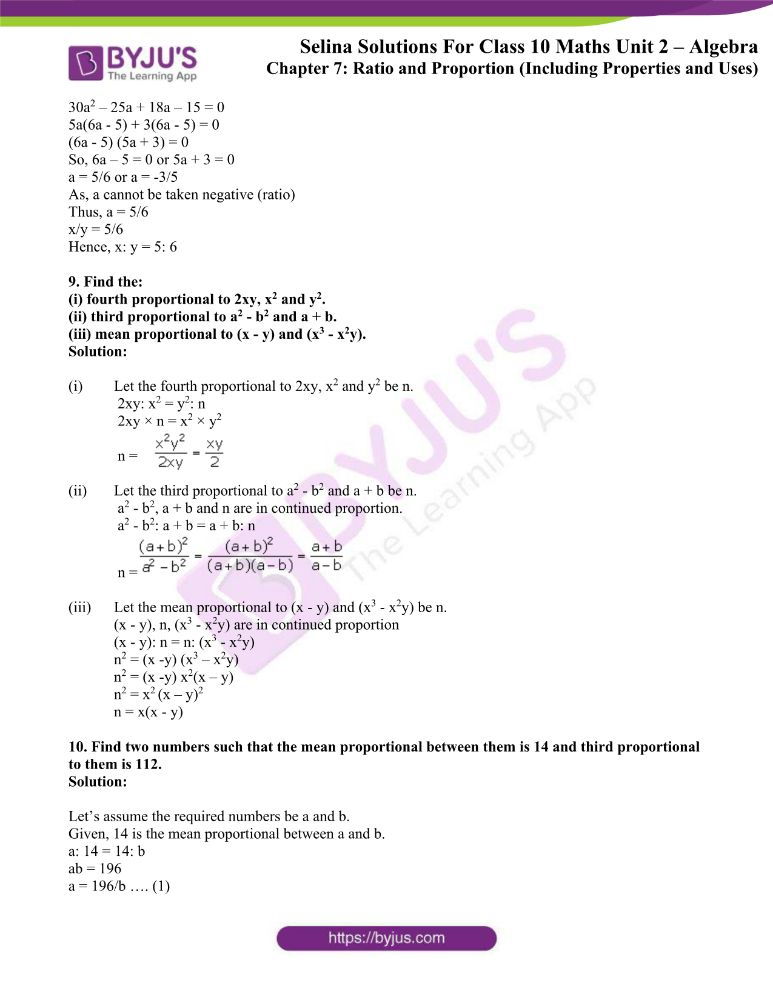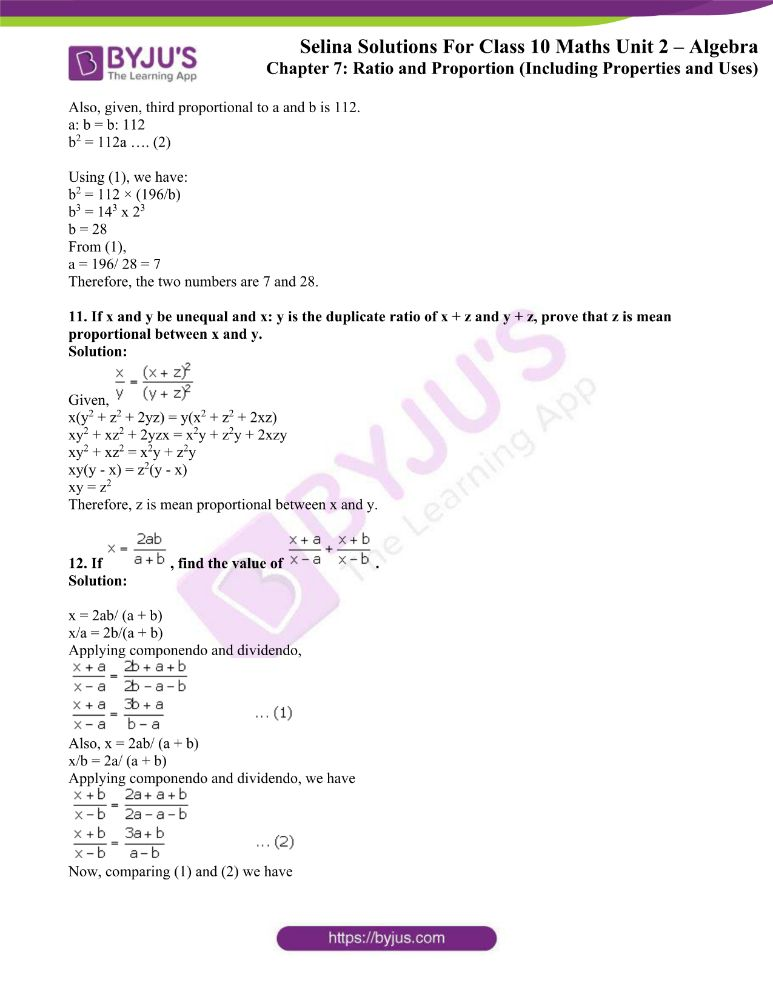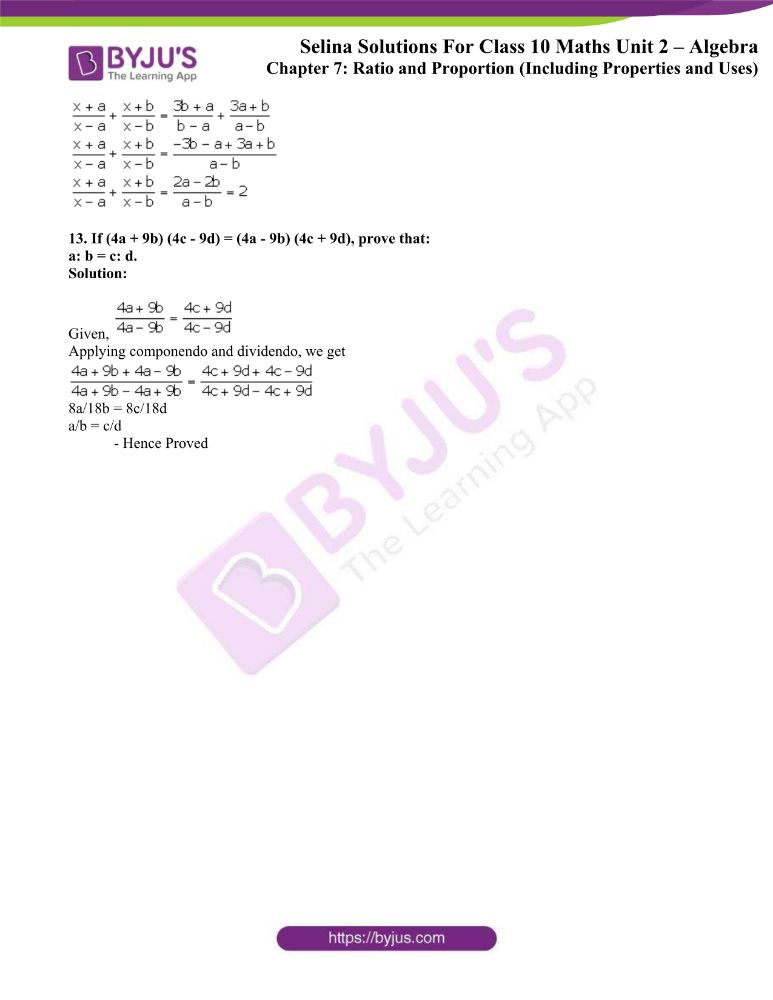### Exercises of Concise Selina Solutions Class 10 Maths Chapter 7 Ratio and Proportions

Exercise 7(A) Solutions

Exercise 7(B) Solutions

Exercise 7(C) Solutions

Exercise 7(D) Solutions

## Access Selina Solutions Concise Maths Class 10 Chapter 7 Ratio and Proportions

Exercise 7(A) Page No: 87

1. If a: b = 5: 3, find: 5a – 3b/ 5a + 3b

Solution:

Given, a: b = 5: 3

So, a/b = 5/3

Now,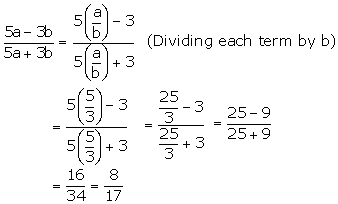2. If x: y = 4: 7, find the value of (3x + 2y): (5x + y).

Solution:

Given, x: y = 4: 7

So, x/y = 4/7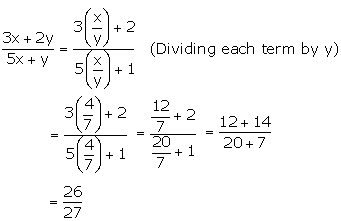3. If a: b = 3: 8, find the value of 4a + 3b/ 6a – b.

Solution:

Given, a: b = 3: 8

So, a/b = 3/8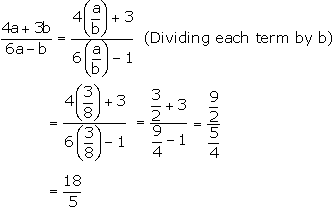4. If (a – b): (a + b) = 1: 11, find the ratio (5a + 4b + 15): (5a – 4b + 3).

Solution:

Given,

(a – b)/ (a + b) = 1/ 11

11a – 11b = a + b

10a = 12b

a/b = 12/10 = 6/5

Now, lets take a = 6k and b = 5k

So,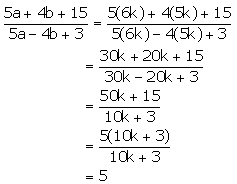Therefore, (5a + 4b + 15): (5a – 4b + 3 = 5: 1

5. Find the number which bears the same ratio to 7/33 that 8/21 does to 4/9.

Solution:

Let consider the required number to be x/y

Now, given that

Ratio of 8/21 to 4/9 = (8/21)/ (4/9) = (8/21) x (9/4) = 6/7

Hence, we have

(x/y)/ (7/33) = 6/7

x/y = (6/7)/ (7/33)

= (6/7) x (7/33)

= 2/11

Therefore, the required number is 2/11.

6.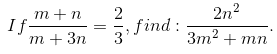Solution:

Given,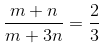3(m + n) = 2(m + 3n)

3m + 3n = 2m + 6n

m = 3n

m/n = 3/1

Now,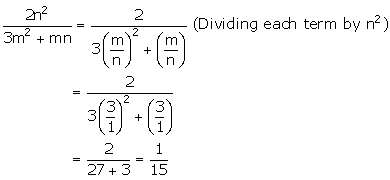7. Find x/y; when x2 + 6y2 = 5xy

Solution:

Given,

x2 + 6y2 = 5xy

Dividing by y2 both side, we have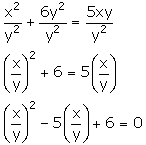Let x/y = a

So,

a2 – 5a + 6 = 0

(a – 2) (a – 3) = 0

a = 2 or a = 3

Therefore, x/y = 2 or 3

8. If the ratio between 8 and 11 is the same as the ratio of 2x – y to x + 2y, find the value of 7x/ 9y.

Solution:

Given,

(2x – y)/ (x + 2y) = 8/11

On cross multiplying, we get

11(2x – y) = 8(x + 2y)

22x – 11y = 8x + 16y

14x = 27y

x/y = 27/14

So,

7x / 9y = (7 x 27)/ (9 x 14) = 3/2

9. Divide Rs 1290 into A, B and C such that A is 2/5 of B and B: C = 4: 3.

Solution:

Given,

B: C = 4: 3 so, B/C = 4/3 ⇒ C = (3/4) B

And, A = (2/5) B

We know that,

A + B + C = Rs 1290

(2/5) B + B + (3/4) B = 1290

Taking L.C.M,

(8B + 20B + 15B)/ 20 = 1290

43B = 1290 x 20

B = 1290 x 20/ 43 = 600

So,

A = (2/5) x 600 = 240

And,

C = (3/4) x 600 = 450

Therefore,

A gets Rs 600, B gets Rs 240 and C gets Rs 450

10. A school has 630 students. The ratio of the number of boys to the number of girls is 3: 2. This ratio changes to 7: 5 after the admission of 90 new students. Find the number of newly admitted boys.

Solution:

Let’s consider the number of boys be 3x.

Then, the number of girls = 2x

⇒ 3x + 2x = 630

5x = 630

x = 126

So, the number of boys = 3x = 3 x 126 = 378

And, number of girls = 2x = 2 x 126 = 252

After admission of 90 new students,

Total number of students = 630 + 90 = 720

Here, let take the number of boys to be 7x

And, the number of girls = 5x

⇒ 7x + 5x = 720

12x = 720

x = 720/12

x = 60

So, the number of boys = 7x = 7 x 60 = 420

And, the number of girls = 5x = 5 x 60 = 300

Therefore, the number of newly admitted boys = 420 – 378 = 42

11. What quantity must be subtracted from each term of the ratio 9: 17 to make it equal to 1: 3?

Solution:

Let x be subtracted from each term of the ratio 9: 17.27 – 3x = 17 – x

10 = 2x

x = 5

Therefore, the required number which should be subtracted is 5.

12. The monthly pocket money of Ravi and Sanjeev are in the ratio 5: 7. Their expenditures are in the ratio 3: 5. If each saves Rs. 80 every month, find their monthly pocket money.

Solution:

Given,

The pocket money of Ravi and Sanjeev are in the ratio 5: 7

So, we can assume the pocket money of Ravi as 5k and that of Sanjeev as 7k.

Also, give that

The expenditure of Ravi and Snajeev are in the ratio 3: 5

So, it can be taken as the expenditure of Ravi as 3m and that of Sanjeev as 5m.

And, each of them saves Rs 80

This can be expressed as below:

5k – 3m = 80 ….. (a)

7k – 5m = 80 …… (b)

Solving equations (a) and (b), we have

k = 40 and m = 40

Therefore, the monthly pocket money of Ravi is Rs 5k = Rs 5 x 40 = Rs 200 and that of Sanjeev is Rs 7k = Rs 7 x 40 = Rs 280.

13. The work done by (x – 2) men in (4x + 1) days and the work done by (4x + 1) men in (2x – 3) days are in the ratio 3: 8. Find the value of x.

Solution:

On assuming that the same amount of work is done one day by all the men and one day work of each man = 1 units, we have

Amount of work done by (x – 2) men in (4x + 1) days

= Amount of work done by (x – 2)*(4x + 1) men in one day

= (x – 2)(4x + 1) units of work

Similarly, we have

Amount of work done by (4x + 1) men in (2x – 3) days

= (4x + 1)*(2x – 3) units of work

Then according to the question, we have8x – 16 = 6x – 9

2x = 7

x = 7/2

14. The bus fare between two cities is increased in the ratio 7: 9. Find the increase in the fare, if:

(i) the original fare is Rs 245;

(ii) the increased fare is Rs 207.

Solution:

From the question we have,

Increased (new) bus fare = (9/7) x original bus fare

(i) We have,

Increased (new) bus fare = 9/7 x Rs 245 = Rs 315

Thus, the increase in fare = Rs 315 – Rs 245 = Rs 70

(ii) Here we have,

Rs 207 = (9/7) x original bus fare

Original bus fare = Rs 207 x 7/9 = Rs 161

Thus, the increase in fare = Rs 207 – Rs 161 = Rs 46

15. By increasing the cost of entry ticket to a fair in the ratio 10: 13, the number of visitors to the fair has decreased in the ratio 6: 5. In what ratio has the total collection increased or decreased?

Solution:

Let’s take the cost of the entry ticket initially and at present to be 10x and 13x respectively.

And let the number of visitors initially and at present be 6y and 5y respectively.

So,

Initially, the total collection = 10x x 6y = 60 xy

And at present, the total collection = 13x x 5y = 65 xy

Hence,

The ratio of total collection = 60 xy: 65 xy = 12: 13

Therefore, it’s seen that the total collection has been increased in the ratio 12: 13.

Exercise 7(B) Page No: 94

1. Find the fourth proportional to:

(i) 1.5, 4.5 and 3.5 (ii) 3a, 6a2 and 2ab2

Solution:

(i) Let’s assume the fourth proportional to 1.5, 4.5 and 3.5 be x.

1.5: 4.5 = 3.5: x

1.5 × x = 3.5 × 4.5

x = (3.5 x 4.5)/ 1.5

x = 10.5

(ii) Let’s assume the fourth proportional to 3a, 6a2 and 2ab2 be x.

3a: 6a2 = 2ab2: x

3a × x = 2ab2 x 6a2

3a × x = 12a3b2

x = 4a2b2

2. Find the third proportional to:

(i)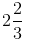and 4 (ii) a – b and a2 – b2

Solution:

(i) Let’s take the third proportional toand 4 be x.

So,, 4, x are in continued proportion.

8/3: 4 = 4: x

(8/3)/ 4 = 4/x

x = 16 x 3/8 = 6

(ii) Let’s take the third proportional to a – b and a2 – b2 be x.

So, a – b, a2 – b2, x are in continued proportion.

a – b: a2 – b2 = a2 – b2: x3. Find the mean proportional between:

(i) 6 + 3√3 and 8 – 4√3

(ii) a – b and a3 – a2b

Solution:

(i) Let the mean proportional between 6 + 3√3 and 8 – 4√3 be x.

So, 6 + 3√3, x and 8 – 4√3 are in continued proportion.

6 + 3√3 : x = x : 8 – 4√3

x × x = (6 + 3√3) (8 – 4√3)

x= 48 + 24√3 – 24√3 – 36

x= 12

x= 2√3

(ii) Let the mean proportional between a – b and a3 – a2b be x.

a – b, x, a3 – a2b are in continued proportion.

a – b: x = x: a3 – a2b

x x x = (a – b) (a3 – a2b)

x2 = (a – b) a2(a – b) = [a(a – b)]2

x = a(a – b)

4. If x + 5 is the mean proportional between x + 2 and x + 9; find the value of x.

Solution:

Given, x + 5 is the mean proportional between x + 2 and x + 9.

So, (x + 2), (x + 5) and (x + 9) are in continued proportion.

(x + 2): (x + 5) = (x + 5): (x + 9)

(x + 2)/ (x + 5) = (x + 5)/ (x + 9)

(x + 5)2 = (x + 2)(x + 9)

x2 + 25 + 10x = x2 + 2x + 9x + 18

25 – 18 = 11x – 10x

x = 7

5. If x2, 4 and 9 are in continued proportion, find x.

Solution:

Given, x2, 4 and 9 are in continued proportion

So, we have

x2/4 = 4/9

x2 = 16/9

Thus, x = 4/3

6. What least number must be added to each of the numbers 6, 15, 20 and 43 to make them proportional?

Solution:

Let assume the number added to be x.

So, (6 + x): (15 + x) :: (20 + x): (43 + x)

(6 + x)/ (15 + x) = (20 + x)/ (43 + x)

(6 + x) (43 + x) = (20 + x) (43 + x)

258 + 6x + 43x + x2 = 300 + 20x = 15x + x2

49x – 35x = 300 – 258

14x = 42

x = 3

Therefore, the required number which should be added is 3.

7. (i) If a, b, c are in continued proportion,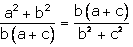Show that:

Solution:

Given,

a, b, c are in continued proportion.

So, we have

a/b = b/c

⇒ b2 = ac

Now,

(a2 + b2) (b2 + c2) = (a2 + ac) (ac + c2) [As b2 = ac]

= a(a + c) c(a + c)

= ac(a + c)2

= b2(a + c)2

(a2 + b2) (b2 + c2) = [b(a + c)][b(a + c)]

Thus, L.H.S = R.H.S– Hence Proved

(ii) If a, b, c are in continued proportion and a(b – c) = 2b, prove that: a – c = 2(a + b)/ a

Solution:

Given,

a, b, c are in continued proportion.

So, we have

a/b = b/c

⇒ b2 = ac

And, given a(b – c) = 2b

ab – ac = 2b

ab – b2 = 2b

ab = 2b + b2

ab = b(2 + b)

a = b + 2

a – b = 2

Now, taking the L.H.S we have

L.H.S = a – c

= a(a – c)/ a [Multiply and divide by a]

= a2 – ac/ a

= a2 – b2/ a

= (a – b) (a + b)/a

= 2(a + b)/a

= R.H.S

– Hence Proved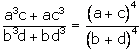(iii) If a/b = c/d, show that:

Solution:

Let’s take a/b = c/d = k

So, a = bk and c = dk

Taking L.H.S,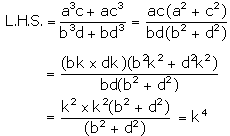Now, taking the R.H.S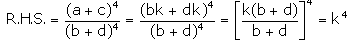Thus, L.H.S = R.H.S

– Hence Proved

8. What least number must be subtracted from each of the numbers 7, 17 and 47 so that the remainders are in continued proportion?

Solution:

Let’s assume the number subtracted to be x.

So, we have

(7 – x): (17 – x):: (17 – x): (47 – x)(7 – x)(17 – x) = (17 – x)2

329 – 47x – 7x + x2 = 289 – 34x + x2

329 – 289 = -34x + 54x

20x = 40

x = 2

Therefore, the required number which must be subtracted is 2.

Exercise 7(C) Page No: 101

1. If a : b = c : d, prove that:

(i) 5a + 7b : 5a – 7b = 5c + 7d : 5c – 7d.

(ii) (9a + 13b) (9c – 13d) = (9c + 13d) (9a – 13b).

(iii) xa + yb : xc + yd = b : d.

Solution:

(i) Given, a/b = c/d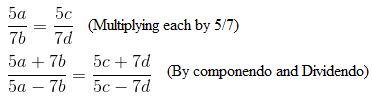(ii) Given, a/b = c/dOn cross-multiplication we have,

(9a + 13b)(9c – 13d) = (9c + 13d)(9a – 13b)

(iii) Given, a/b = c/d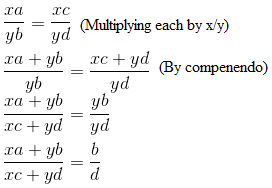– Hence Proved

2. If a : b = c : d, prove that:

(6a + 7b) (3c – 4d) = (6c + 7d) (3a – 4b).

Solution:

Given, a/b = c/d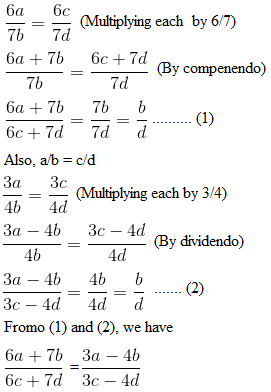(6a + 7b)(3c – 4d) = (3a – 4b)(6c + 7d)

– Hence Proved

3. Given, a/b = c/d, prove that:

(3a – 5b)/ (3a + 5b) = (3c – 5d)(3c + 5d)

Solution: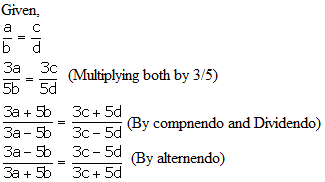4. If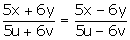;

Then prove that x: y = u: v

Solution: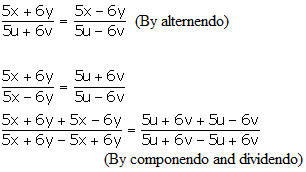10x/ 12y = 10u/ 12v

Thus,

x/y = u/v ⇒ x: y = u: v

5. If (7a + 8b) (7c – 8d) = (7a – 8b) (7c + 8d);

Prove that a: b = c: d

Solution:

The given can the rewritten as,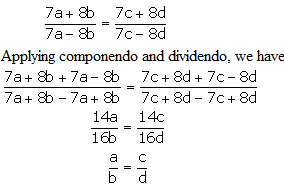6. (i) If x = 6ab/ (a + b), find the value of: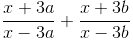Solution:

Given, x = 6ab/ (a + b)

⇒ x/3a = 2b/ a + b

Now, applying componendo and dividendo we have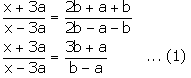Again, x = 6ab/ (a + b)

⇒ x/3b = 2a/ a + b

Now, applying componendo and dividendo we have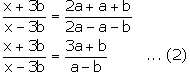From (1) and (2), we get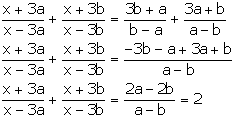(ii) If a = 4√6/ (√2 + √3), find the value of: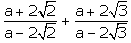Solution:

Given, a = 4√6/ (√2 + √3)

a/2√2 = 2√3/ (√2 + √3)

Now, applying componendo and dividendo we have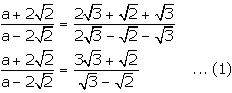Again, a = 4√6/ (√2 + √3)

a/2√3 = 2√2/ (√2 + √3)

Now, applying componendo and dividendo we have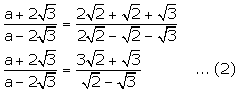From (1) and (2), we have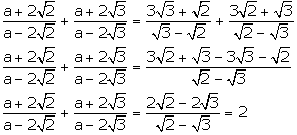7. If (a + b + c + d) (a – b – c + d) = (a + b – c – d) (a – b + c – d), prove that a: b = c: d.

Solution:

Rewriting the given, we have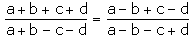Now, applying componendo and dividendo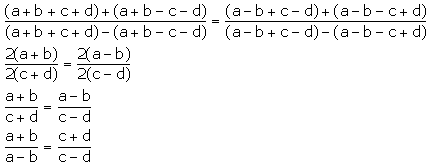Applying componendo and dividendo again, we get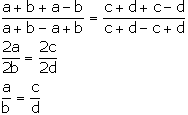– Hence Proved

Exercise 7(D) Page No: 102

1. If a: b = 3: 5, find:

(10a + 3b): (5a + 2b)

Solution:

Given, a/b = 3/5

(10a + 3b)/ (5a + 2b)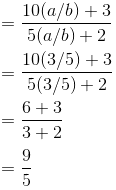2. If 5x + 6y: 8x + 5y = 8: 9, find x: y.

Solution: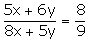Given,

On cross multiplying, we get

45x + 54y = 64x + 40y

14y = 19x

Thus,

x/y = 14/19

3. If (3x – 4y): (2x – 3y) = (5x – 6y): (4x – 5y), find x: y.

Solution:

Given, (3x – 4y): (2x – 3y) = (5x – 6y): (4x – 5y)

This can be rewritten as,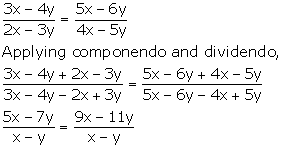5x – 7y = 9x – 11y

4y = 4x

x/y = 1/1

Thus,

x: y = 1: 1

4. Find the:

(i) duplicate ratio of 2√2: 3√5

(ii) triplicate ratio of 2a: 3b

(iii) sub-duplicate ratio of 9x2a: 25y6b2

(iv) sub-triplicate ratio of 216: 343

(v) reciprocal ratio of 3: 5

(vi) ratio compounded of the duplicate ratio of 5: 6, the reciprocal ratio of 25: 42 and the sub-duplicate ratio of 36: 49.

Solution:

(i) Duplicate ratio of 2√2: 3√5 = (2√2)2: (3√5)2 = 8: 45

(ii) Triplicate ratio of 2a: 3b = (2a)3: (3b)3 = 8a3: 27b3

(iii) Sub-duplicate ratio of 9x2a4: 25y6b2 = √(9x2a4): √(25y6b2) = 3xa2: 5y3b

(iv) Sub-triplicate ratio of 216: 343 = (216)1/3: (343)1/3 = 6: 7

(v) Reciprocal ratio of 3: 5 = 5: 3

(vi) Duplicate ratio of 5: 6 = 25: 36

Reciprocal ratio of 25: 42 = 42: 25

Sub-duplicate ratio of 36: 49 = 6: 7

Required compound ratio =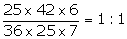5. Find the value of x, if:

(i) (2x + 3): (5x – 38) is the duplicate ratio of √5: √6.

(ii) (2x + 1): (3x + 13) is the sub-duplicate ratio of 9: 25.

(iii) (3x – 7): (4x + 3) is the sub-triplicate ratio of 8: 27.

Solution:

(i) (2x + 3): (5x – 38) is the duplicate ratio of √5: √6

And, the duplicate ratio of √5: √6 = 5: 6

So,

(2x + 3)/ (5x – 38) = 5/6

12x + 18 = 25x – 190

25x – 12x = 190 + 18

13x = 208

x = 208/13 = 16

(ii) (2x + 1): (3x + 13) is the sub-duplicate ratio of 9: 25

Then the sub-duplicate ratio of 9: 25 = 3: 5

(2x + 1)/ (3x + 13) = 3/5

10x + 5 = 9x + 39

x = 34

(iii) (3x – 7): (4x + 3) is the sub-triplicate ratio of 8: 27

And the sub-triplicate ratio of 8: 27 = 2: 3

(3x – 7)/ (4x + 3) = 2/3

9x – 8x = 6 + 21

x = 27

6. What quantity must be added to each term of the ratio x: y so that it may become equal to c: d?

Solution:

Let’s assume the required quantity which has to be added be p.

So, we havedx + pd = cy + cp

pd – cp = cy – dx

p(d – c) = cy – dx

p = cy –dx/ (d – c)

7. A woman reduces her weight in the ratio 7: 5. What does her weight become if originally it was 84 kg?

Solution:

Let’s consider the woman’s reduced weight as x.

Given, the original weight = 84 kg

So, we have

84: x = 7: 5

84/x = 7/5

84 x 5 = 7x

x = (84 x 5)/ 7

x = 60

Therefore, the reduced weight of the woman is 60 kg.

8. If 15(2x2 – y2) = 7xy, find x: y; if x and y both are positive.

Solution:

15(2x2 – y2) = 7xyLet’s take the substitution as x/y = a

2a – 1/a = 7/15

(2a2 – 1)/ a = 7/15

30a2 – 15 = 7a

30a2 – 7a – 15 = 0

30a2 – 25a + 18a – 15 = 0

5a(6a – 5) + 3(6a – 5) = 0

(6a – 5) (5a + 3) = 0

So, 6a – 5 = 0 or 5a + 3 = 0

a = 5/6 or a = -3/5

As, a cannot be taken negative (ratio)

Thus, a = 5/6

x/y = 5/6

Hence, x: y = 5: 6

9. Find the:

(i) fourth proportional to 2xy, x2 and y2.

(ii) third proportional to a2 – b2 and a + b.

(iii) mean proportional to (x – y) and (x3 – x2y).

Solution:

(i) Let the fourth proportional to 2xy, x2 and y2 be n.

2xy: x2 = y2: n

2xy × n = x2 × y2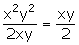n =

(ii) Let the third proportional to a2 – b2 and a + b be n.

a2 – b2, a + b and n are in continued proportion.

a2 – b2: a + b = a + b: n

n =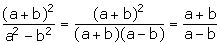(iii) Let the mean proportional to (x – y) and (x3 – x2y) be n.

(x – y), n, (x3 – x2y) are in continued proportion

(x – y): n = n: (x3 – x2y)

n2 = (x -y) (x3 – x2y)

n2 = (x -y) x2(x – y)

n2 = x2 (x – y)2

n = x(x – y)

10. Find two numbers such that the mean proportional between them is 14 and third proportional to them is 112.

Solution:

Let’s assume the required numbers be a and b.

Given, 14 is the mean proportional between a and b.

a: 14 = 14: b

ab = 196

a = 196/b …. (1)

Also, given, third proportional to a and b is 112.

a: b = b: 112

b2 = 112a …. (2)

Using (1), we have:

b2 = 112 × (196/b)

b3 = 143 x 23

b = 28

From (1),

a = 196/ 28 = 7

Therefore, the two numbers are 7 and 28.

11. If x and y be unequal and x: y is the duplicate ratio of x + z and y + z, prove that z is mean proportional between x and y.

Solution:

Given,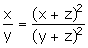x(y2 + z2 + 2yz) = y(x2 + z2 + 2xz)

xy2 + xz2 + 2yzx = x2y + z2y + 2xzy

xy2 + xz2 = x2y + z2y

xy(y – x) = z2(y – x)

xy = z2

Therefore, z is mean proportional between x and y.

12. If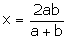, find the value of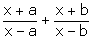.

Solution:

x = 2ab/ (a + b)

x/a = 2b/(a + b)

Applying componendo and dividendo,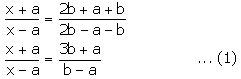Also, x = 2ab/ (a + b)

x/b = 2a/ (a + b)

Applying componendo and dividendo, we have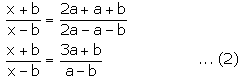Now, comparing (1) and (2) we have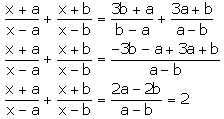13. If (4a + 9b) (4c – 9d) = (4a – 9b) (4c + 9d), prove that:

a: b = c: d.

Solution:

Given,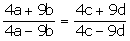Applying componendo and dividendo, we get8a/18b = 8c/18d

a/b = c/d

– Hence Proved

The given solutions are as per the 2019-20 Concise Selina textbook. The Selina Solutions for the academic year 2020-21 will be updated soon.